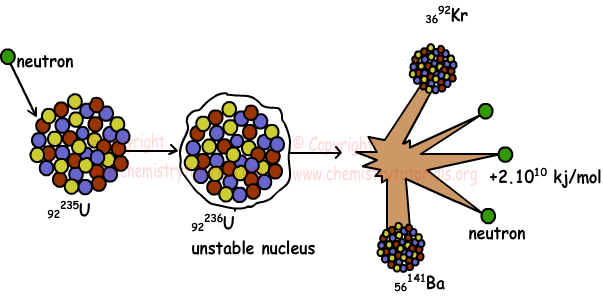## Artificial Radioactivity Fission and Fusion

Two artificial nuclear reactions were done by Rutherford. In this reactions, Rutherford use α decay to convert 417N to 817O.

417N + 24He → 817O + 11H

817O is not radioactive element.First artificial radioactive nucleus is 1530P and it is produced by alpha decay of 1327Al.

1327Al + 24He → 1530P + 01n

1530P → 1430Si + β+

In these reactions, 1530P is radioactive nucleus, and it is converted to 1430Si by positron decay. Neutrons 01n, protons 11H, deuterium 12H are used in artificial nuclear reactions. Now we explain to important artificial nuclear reactions fission and fusion.

1. Nuclear Fission:

Nuclear fission is a nuclear reaction in which nucleus of atom split into smaller particles. Nucleus having mass number larger than 200 does neutron decay and split into elements having smaller mass numbers.

Example:

92235U + 01n → 56141Ba + 3692Kr + 301n + Energy

Nuclear fission is an exothermic reaction and excess amount of energy is released. By the help of these reactions, now energy is produced in nuclear power plants. Picture given below shows fission of uranium;2. Nuclear Fusion:

More than one nucleus, having small atomic masses, are combined to form heavier new nucleus. Nuclear fusion is also exothermic reactions and energy released in these reactions are larger than energy released in fission reactions. On the contrary, there must be large amount of energy to start fusion reactions.In hydrogen bomb we see fusion reactions.

Example:

411H → 24He + 2β+

12H + 13H → 24He +01n

Example: Which ones of the following statements are true for nuclear reactions?

I. Sum of mass number is conserved

II. Mass loss is not important

III. Structure of nucleus can change

Solution:

In nuclear reactions, sum of number of protons and neutrons is always conserved. However, in nuclear reactions mass is not conserved. Lost mass is converted to energy, so amount of mass is important. In nuclear reactions one atom can converted to another atom. I and III are true.

Example: Which ones of the following statements are true for following reaction;

49Be + 13H → 511B + 01n

I. It is fusion reaction

II. It is natural nuclear reaction

III. Number of neutron is conserved

Solution:

49Be and 13H are joined to form 511B. Thus, this is fusion reaction in other words artificial nuclear reaction. If we write number of neutrons in both sides of reaction;

49Be + 13H → 511B + 01n

5+2=6+1

7=7 Number of neutrons is conserved in this reaction.

Example: Find Z in the reaction given below.

a32X + 24He → (a+2)35Y + Z

Solution:

a32X + 24He → (a+2)35Y + bcZ

conservation of charge;

a+2=(a+2)+b

b=0

Conservation of mass;

32+4=35+c

c=1

Thus, Z is neutron 01n.

Nuclear Chemistry (Radioactivity) Exams and  Problem Solutions

Related